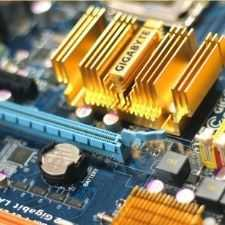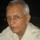Electronics
Community of Electronics Engineers to discuss all concepts, topics, questions and doubts.
579 Members
Join this group to post and comment.sreekant • Dec 3, 2008

# Why is the power factor needed in ac circuits and hence its correction?

why is the power factor needed in ac circuits and hence its correction?-explain to a beginner.:sshhh:avm • Dec 3, 2008
Power factor is actually a factor which decides how much power can be taken in at the receiving end. All the power from the sending end will not reach the receiving end. It is dependent on the resistance and impedance.Fahad Bhatti • Dec 9, 2008
In a purely resistive network, the voltage and current are always in phase, means both sine waves rise and fall at the same time, But in practice the household and industrial loads are inductive such as motors, fans etc. This makes the current lag the voltage and the product of VI = POWER is no more valid.

More the load is inductive more the current lags... which must be corrected,
This correction is called Power Factor Correction.

In above discussed case of inductive load the power factor correction should be made by adding a capacitor which would cancel the effect of indcutor.

Thus power factor correction is all about bringing Voltage and Current in phase.ashuashi • Jan 11, 2009
sreekant
why is the power factor needed in ac circuits and hence its correction?-explain to a beginner.:sshhh:
Adding to above post...

Poor power factor may cost you more. As in some places Electricity board charge on KVAH. KWh is independent of power factor. Thus poor power factor make KVAH more & hence you pay more. If you keep good p.f. you pay less as well as Electricity Board provides rebate also.To make things simple,losses in AC is more than DC.power factor is one of the ways to monitor the loss,AC is preferred over DC since it is cheaper and easier to generate compare to DCashuashi • Jan 12, 2009
sauravgoswami
To make things simple,losses in AC is more than DC.power factor is one of the ways to monitor the loss,AC is preferred over DC since it is cheaper and easier to generate compare to DC
Hi Saurav,

Does this mean... We improve losses by improving power factor, adding capacitor bank to our system? 😲nothing as such,but ofcourse we check the health of our system and maintain it at a right time,thus decreasing the losses.pls correct if i am wrongashuashi • Jan 12, 2009
sauravgoswami
nothing as such,but ofcourse we check the health of our system and maintain it at a right time,thus decreasing the losses.pls correct if i am wrong
No doubt our system gets improved with good power factor. But I have a doubt on improving losses... bcoz if we improve P.F., we install capacitor banks which draw extra current from the system.

Please also correct me if I am wrong...ashuashi
Hi Saurav,

Does this mean... We improve losses by improving power factor, adding capacitor bank to our system? 😲
We can minimize reactive losses by improving power factor.
And we can do that by adding capacitor bank of course.
But we generaly choose a moderate value of power factor becuase of cost associated with adding capacitors.
We can also improve power factor using condensers or overexcited induction motors.avm • Jan 12, 2009
The actual amount of power generated is the apparant power. But due to the inductive effect of the circuit the power delivered at the receiving end (load) will be a percentage of the apparent power. So the power factor is actually an indication of the percentage utilization of the generated power. If we say that the powerfactor of a circuit is 0.8, then the amount of utilization of the apparent power is 80%.

........................................ashuashi • Jan 12, 2009
frodo.rok
We can minimize reactive losses by improving power factor.
And we can do that by adding capacitor bank of course.
But we generaly choose a moderate value of power factor becuase of cost associated with adding capacitors.
We can also improve power factor using condensers or overexcited induction motors.
May I beg your pardon please... Condensers are capacitors only. Yes we can also improve power factor by over-excited motors but not induction -- They are synchronous motors.

correct me if I am wrongvasanthmbs • Jan 14, 2009
power factor is angle difference between voltage and current .... in pure resistive network voltage is in phase with current ... but in inductive or capacitive circuit voltage lags and leads ... so that there is change in load supply... if frequency changes there might be heavy losses ... so that we are maintaing power factor in correct range. pure resistive circuit is nothing but DC circuit.. in dc circuit there is no frequency.. hence dont bother about power factor in dc circuits.....yeah but how can synchronous motors help in reducing this angle or maintaining frequency to compensate for power loss!!!vasanthmbs • Jan 15, 2009
synchronous motor will not control power factor ........ it is totally depends on transmission and distribution at last to load......ashuashi • Jan 15, 2009
vasanthmbs
synchronous motor will not control power factor ........ it is totally depends on transmission and distribution at last to load......
If Synchronous motor runs in over excited condition, it draws leading power factor from the system. Hence if there are synchronous motors installed, can be used to improve the p.f. if run in over excited condition.vasanthmbs • Jan 15, 2009
yes at excited condition it emits leading power factor........ bcoz at extreme power it act as condenser (capacitor) ...... but most of the loads are inductive in nature ...... hence it is balanced .. if it is not satisfied we can use inductor bank.... and also vice versallourdujeyaraj • Aug 1, 2009
why is the power factor coming in picture in ac circuitskashish0711 • Aug 2, 2009
Power factor tells us about the real power being used in a circuit. We do pf correction to reduce the reactive power(KVAR) of the circuit. It gives us many benefits such as, it reduces the operating cost, reduces losses in a circuit, better efficiency. Poor power factor is caused by inductive loads.2592sanju • Jul 25, 2011
PF is reduced by inductors and caps so whats the real use of inductors and capspraba230890 • Jul 25, 2011
No way!!!!!! PF is reduced by inductors but not capacitors. Always capacitors were a good friends to PF(which boosts the power factor).
2592sanju
PF is reduced by inductors and caps so whats the real use of inductors and capspraba230890 • Jul 25, 2011
Every electrical engineer must be atleast heard of one of the power factor definition - PF is the cosine of the angle between current and voltage. "Angle" - what it mean by?

As we know the A.C supply that we getting to our home are periodic and sinusoidal. Because of it's periodicity the voltage and current can be drawn in graph with respect to degrees instead of time(0-360deg). We will be expecting that both the voltage and current's sinusoidal variation should be same all over the supply period.

This won't happen at all due to the presence of some inductive(mostly) and capacitive(rarely) characteristics of the conductors. The inductors always deny the current flow(lenz law) which makes this current lagging nature of power supply and this is why we need capacitors which 'delays the voltage applied but not current' helps us to make them flow synchronously. This is why we always keep on calculating the value of capacitance of the capacitive banks needed to add at high inductive load points to improve PF.

Finally i'm answering your question. As we are keep track on voltage and current in degrees instead of time the time difference between the voltage and current waveform due to inductor/capacitors becomes the degree value. We got the degree now, which is the angle between voltage and current and we are calculating the power factor by cosining(i mean the cos (angle)) it. And in D.C, afterall it is non varying why we need to even think of PF.
llourdujeyaraj
why is the power factor coming in picture in ac circuitssaketsingh • Aug 5, 2011
Well let me clear u out we dont use capacitor bank. We use capacitor or condensor just to compensate the lagging of the current due to inductive in the line that is what is called as "pie" structure of the "T" structure where the capacitor are arrange in parallel.

Capacitor banks are used for the storage of the charges to create high voltage. etc...
power factor is noting but the active power/apparent power.
where apparent power = (active power + reactive power).